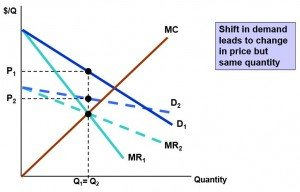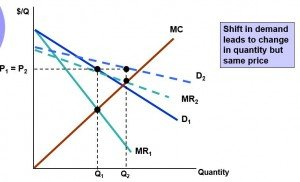Select Page

A Monopolistic market has no Supply curve

The reason behind it the NO SUPPLY CURVE is that the monopolist output decision depends not only on Marginal cost but also on the Shape of Demand Curve. Note that the shape of demand curve do not tell us Price and Quantity that reaches to a competitive supply curve. Instead, shifts in demand curve can lead to

1. Changes in Price with no change in Output

2. Change in output with no change in Price

3. Changes in Price and Output both### Graphically

The Demand Curve D1 shifts to D2. And the new marginal revenue curve MR2 intersects marginal cost at the same point as old marginal revenue curve. Therefore, the Profit maximizing output remains the same although the Price shifts from P1 to P2(downwards)In this case, the marginal revenue MR2 intersects the Marginal cost curve at a higher output level Q2. But we can also from the curve that the demand is elastic so the price remains the same.

Instead of a competitive industry which supplies a specific quantity at every price, a monopoly does not have any such relationship and it dependent over the shifts in demand.

Shares